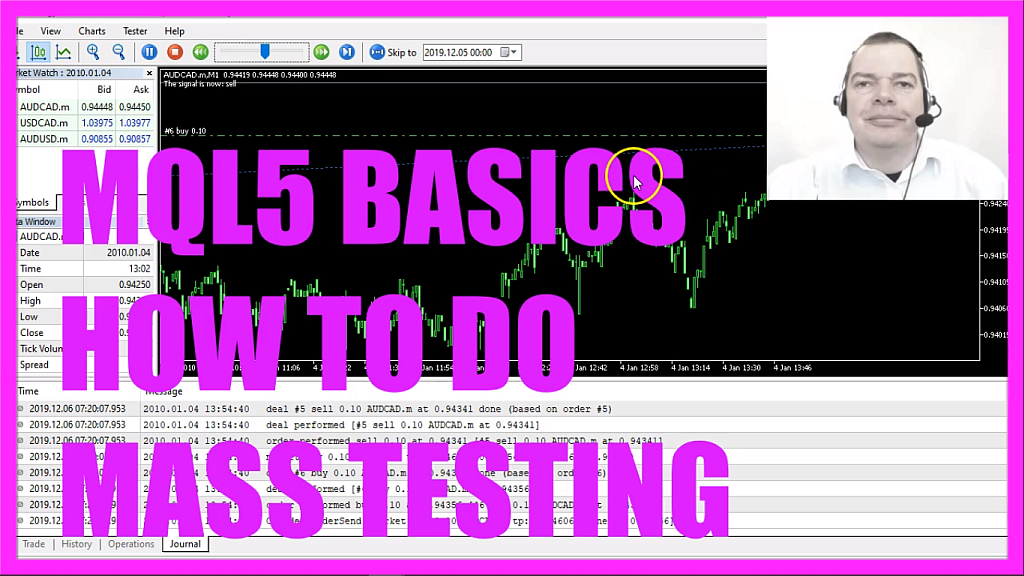In this video we are going to do mass testing with a random entry, we use buy and sell signals here, those are created by a random generator.
You might be familiar with this kind of testing, that’s the visual mode but it’s also possible to remove the hook here.
Let’s do a quick test here for the year twenty eighteen, click on start, here is the graph, it only took a few seconds. In this case, we made a profit of three hundred and one dollars and forty-eight cents.
Let’s repeat it with the same settings, this time we lost twenty-four dollars and seventy-seven cents, let’s do one more.
And this was also a loss, we lost one hundred ninety-three dollars, so for the same time span we have different results each time because we are using random generated entries and now we would like to do a mass test, so let’s go to settings, this is the source code from the last expert advisor that we have created in the video before and here we have an input value, it’s an integer called mass test and this is an external variable that can be changed without changing the source code and that’s exactly what we need because this makes it possible to set the values for the mass test here but right now it doesn’t work, we can’t change anything because before we do that we need to enable the optimization here.
So let’s click on optimization and choose fast generic based algorithm, now we go back to inputs and this time we can change the value here, so let’s click on start and now you should see some optimization results on this tab here. We did fifteen tests in a few seconds, each value here is represented by a dot here and all the dots that are above this mark here stand for a profit, so I would say we had more profitable results than losses but we want to find out exactly.
So let’s right-click here, select export to xml, I will call this file masstest dot xml, save it, this is what it looks like in your web browser, not very spectacular but it’s also possible to open it in a spreadsheet calculation.
Here we are, this is the profit column and now we can calculate the end result here and we made an overall profit of two thousand nine hundred and twenty-one dollars even with some minor losses here that could still be random so you will be able to do a hundred or even a thousand tests with this kind of mass testing and that is the power of automated trading because discretionary traders only see this kind of information and they see it much slower but now you know how to do mass test with random based entries in a very short time and you can do it yourself with a few lines of mql5 code.